### Algorithm Fundamentals

Many algorithms can be described with just conditionals, loops, and numeric values that can be stored in assignable variables, like this:

Assignable variables help algorithms juggle lots of different values. But if you want to think about algorithms that work on significant amounts of data, like all the words in a book or all the files on your computer, you've got to work with arrays.Understanding how arrays work is essential for understanding the most interesting algorithms and data structures.

# Arrays

You’ve already learned that an assignable is like a single location that can store a value. An assignable is like a tabletop: you can store the number 6 by having 6 plums on a table.

An array is a sequence of locations that can store values. You can think of an array as a sequence of cubbyholes, where each cubbyhole can store a number.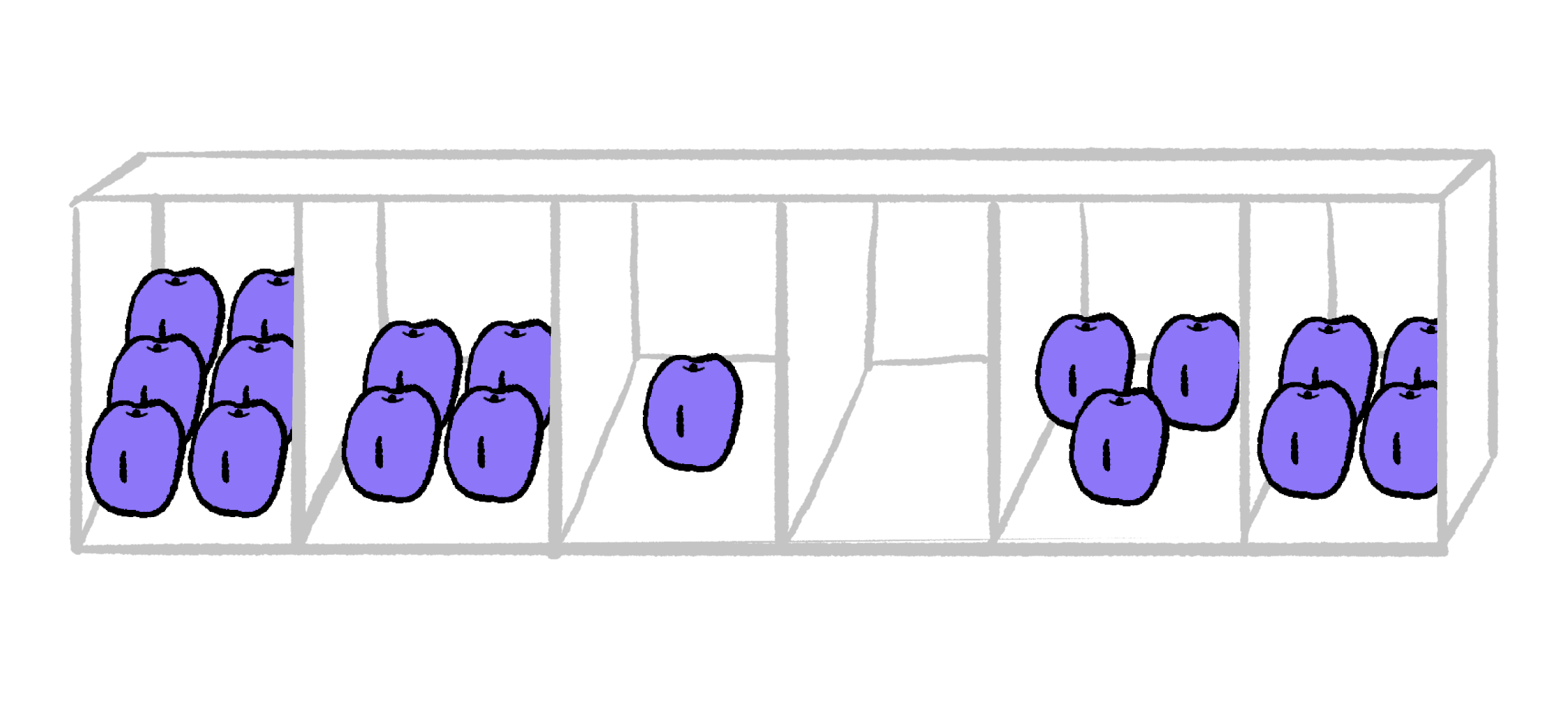# Arrays

Each location in an array is given an index, that location's "address" within the array. In this course, we’ll label the first location of an array with the index 1, the second location with the index 2, and so on.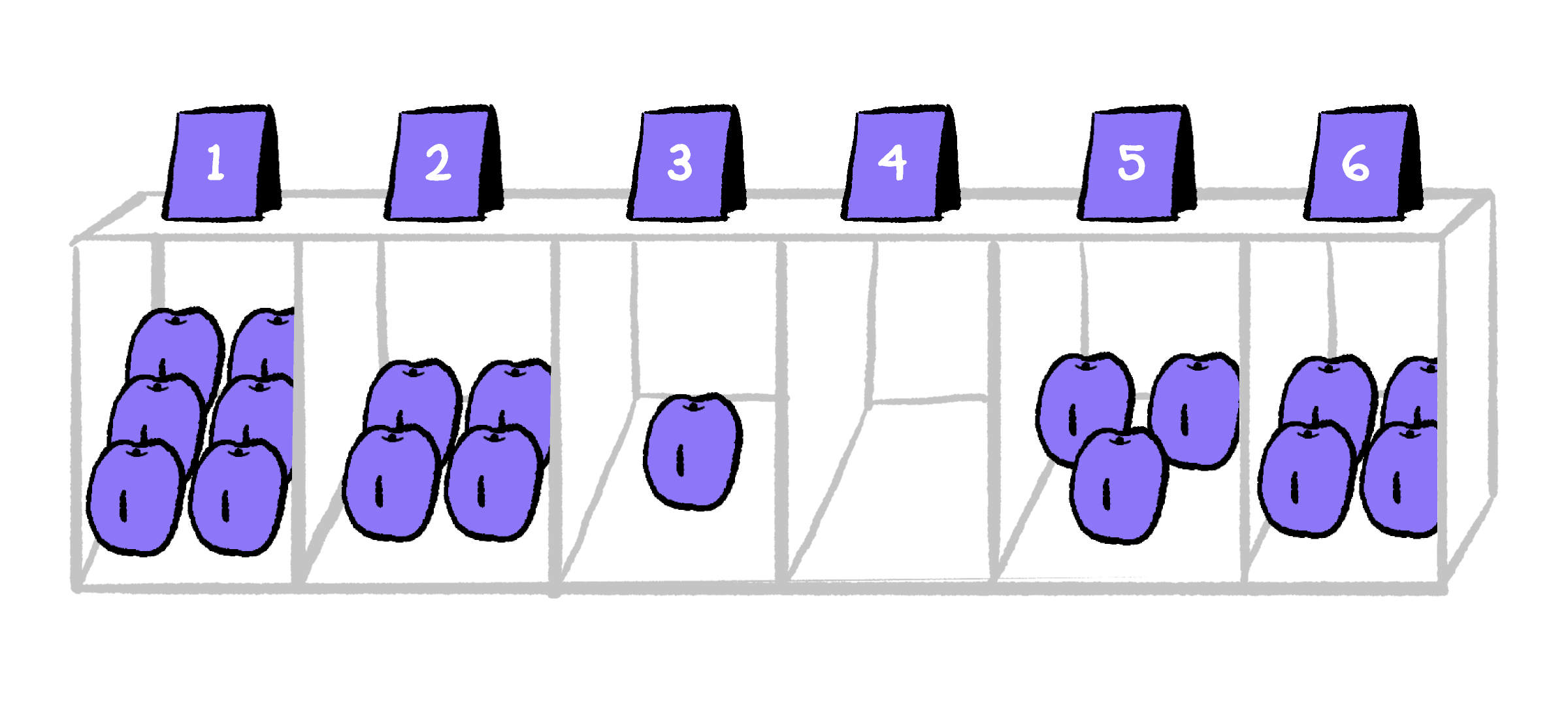If A is the name of the array above, then you refer to the first location — the location with index 1 — as A. The location with index 2 is A.

What are the contents of A in the array above?

# Arrays

Rather than piles of plums, a computer scientist will usually represent arrays and their contents more abstractly. Arrays in computer science are usually represented like this: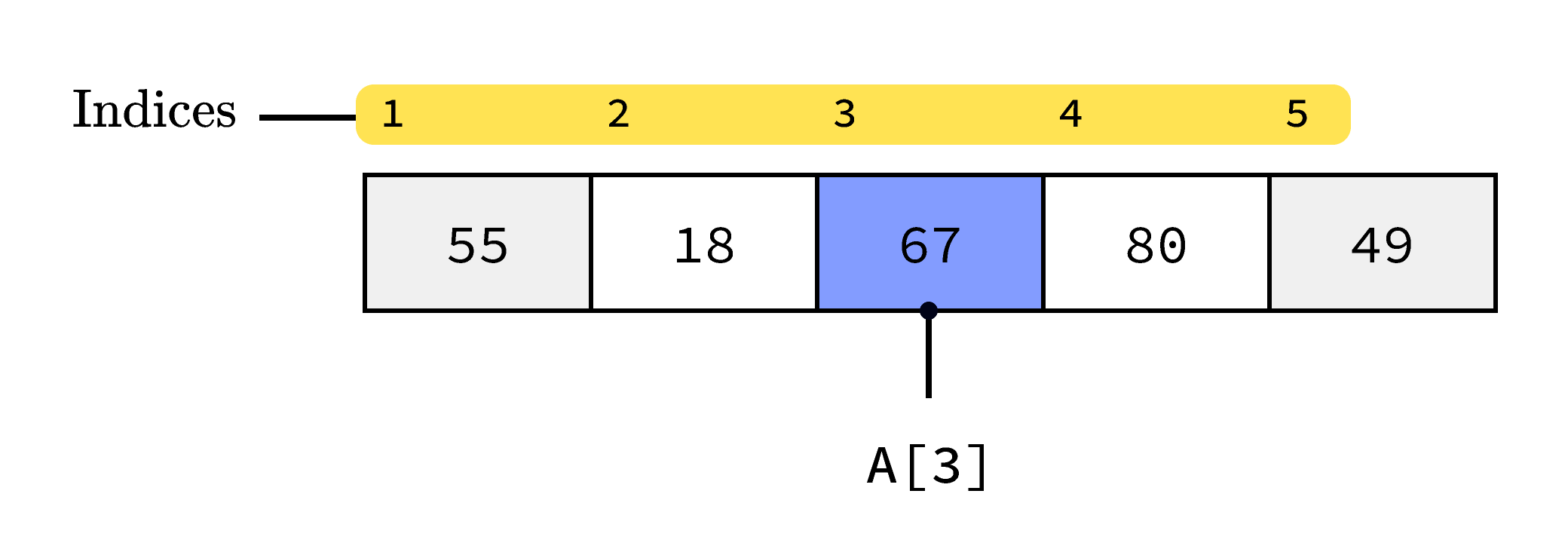Select and arrange some of the commands below so that, if the commands are run on the array A shown above, the resulting array will be a sorted array. You won’t use every line.

# Arrays

Arrays can have different lengths. An array may store three values, five values like the array below, or billions of values!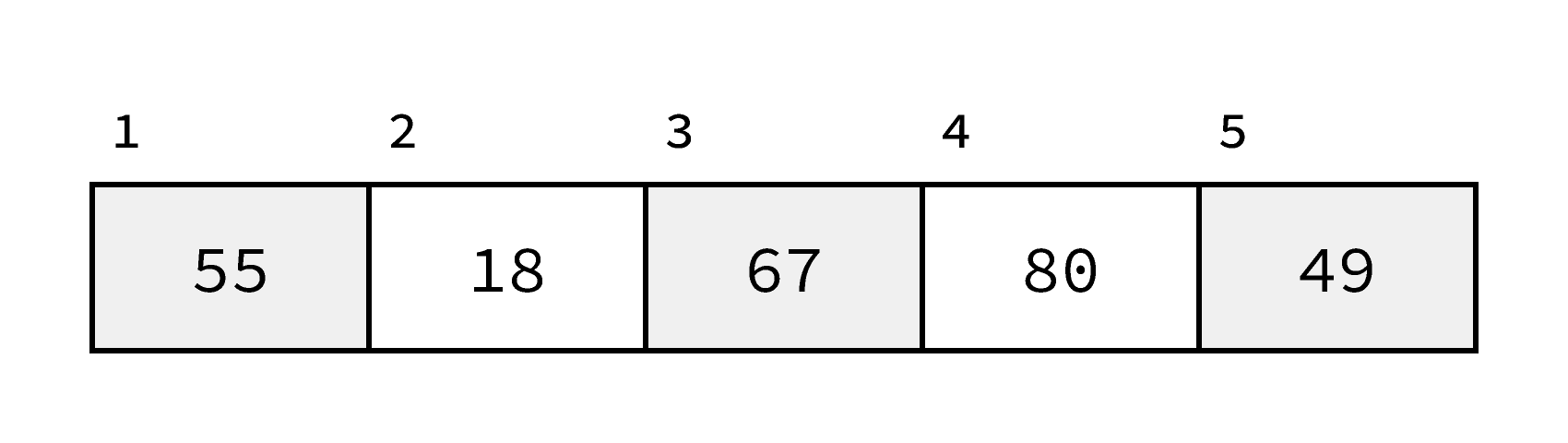If an array has only one accessible location, what is the index of that one location?

# Arrays

Algorithms that use arrays will usually need to work on arrays of all different lengths. Repetition instructions that check the length of the array are crucial for writing these algorithms.

The algorithm below stores 5 at every position in the array, regardless of what was there before.

# Arrays

Try to think through what happens in the following algorithm. It might be helpful to imagine a very short array of size 2 or 3.

What will this algorithm do?

# Arrays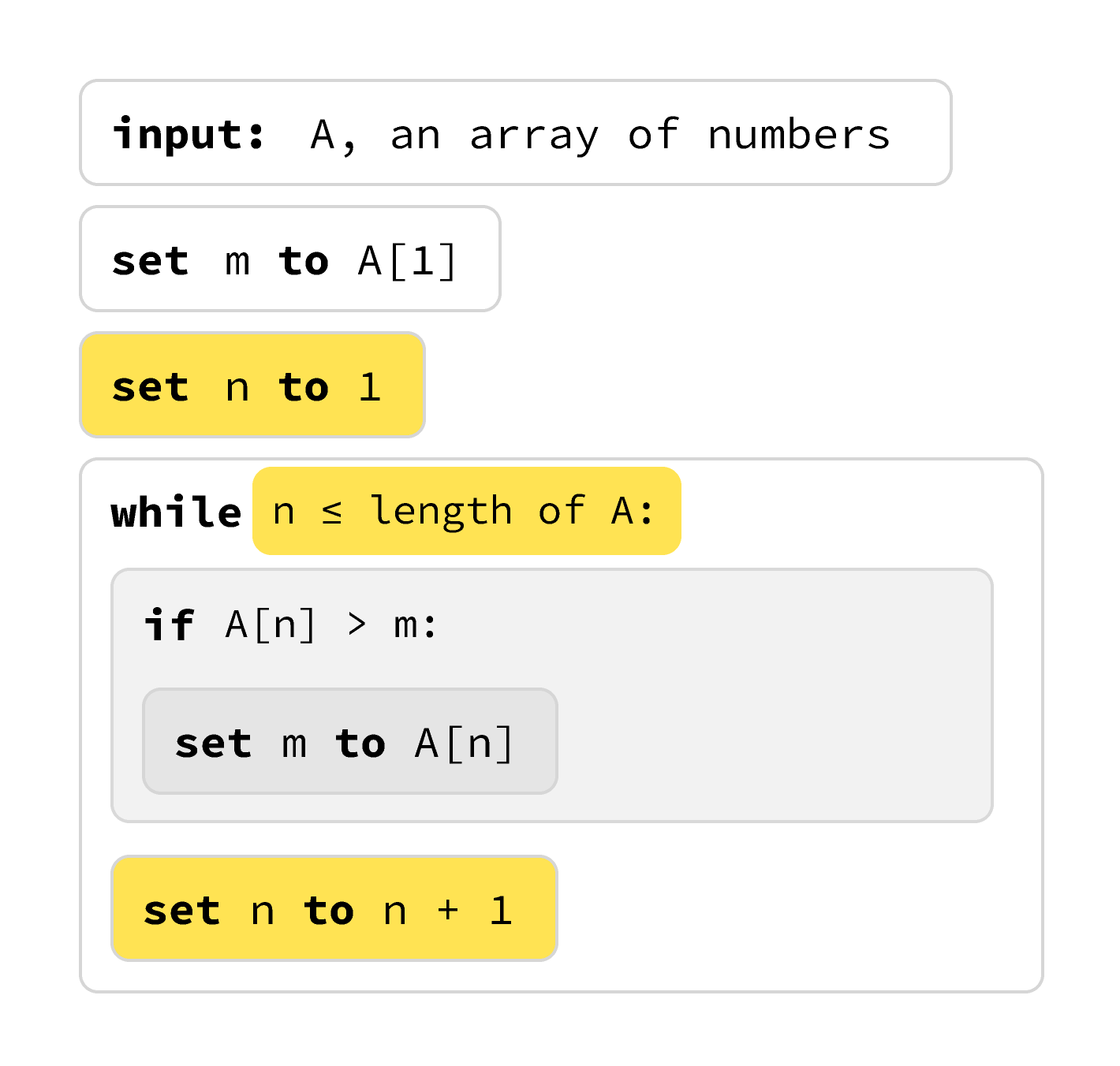This pattern you just saw is everywhere when you're dealing with loops: you need a repetition instruction with an assignable variable having the value of 1, then 2, then 3... all the way to the last valid array index, which is equal to the length of the array.

It’s important enough that it’s worth having a simplified way of writing it down in your pseudocode vocabulary.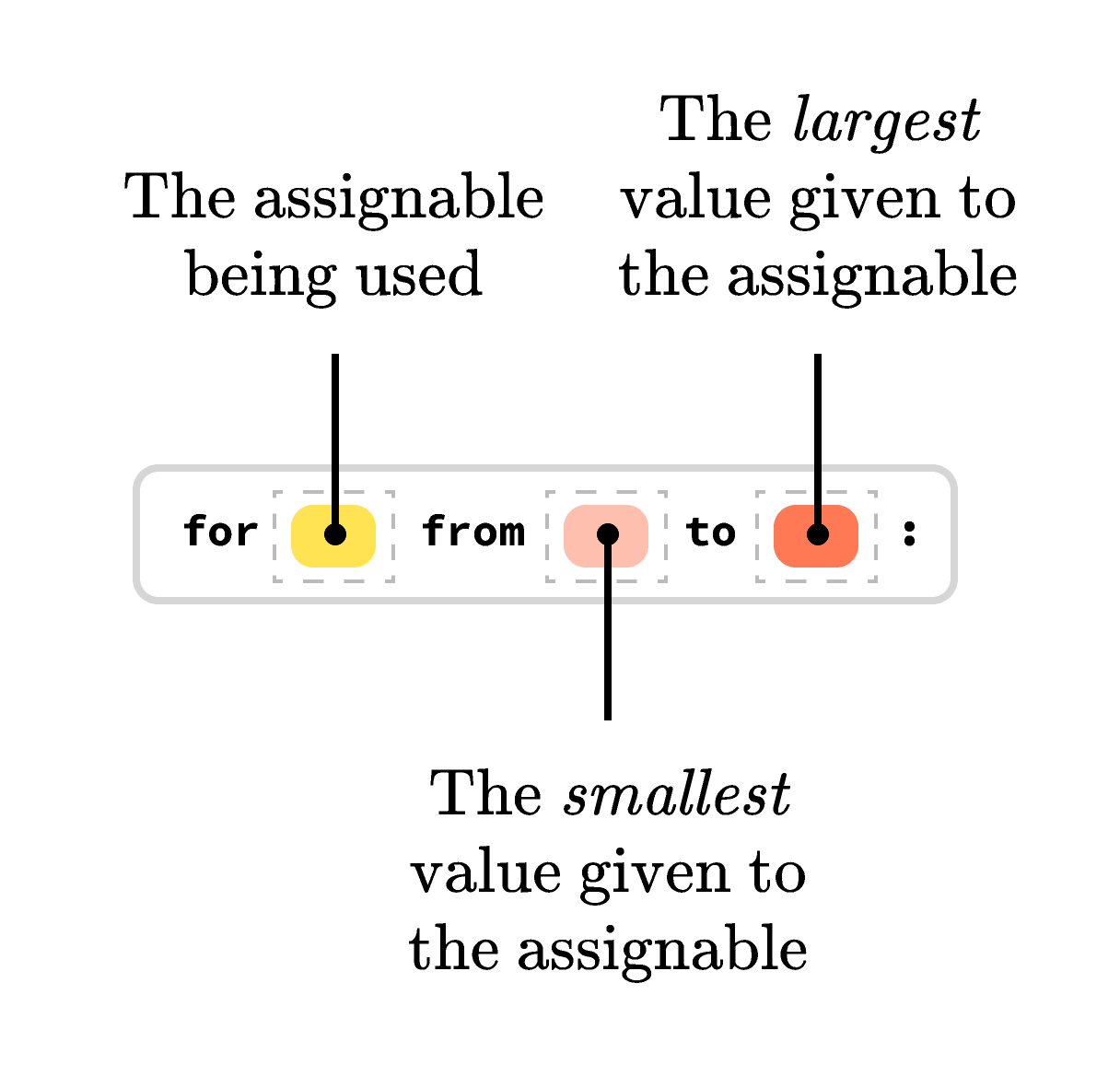The repetition instruction for is a good fit. Instead of having a test, a for instruction lists an assignable, the smallest value that will be put in the assignable (when the commands inside are run for the first time), and the greatest value that will be put in the assignable (when the commands inside are run for the last time).

# ArraysHere's that algorithm for finding the maximum element in an array with a while instruction again:

# Arrays

Using the for repetition instruction is a natural fit with arrays.

# Arrays

Construct an algorithm so that, when the algorithm finishes, the assignable variable total will contain the sum of all the numbers in A.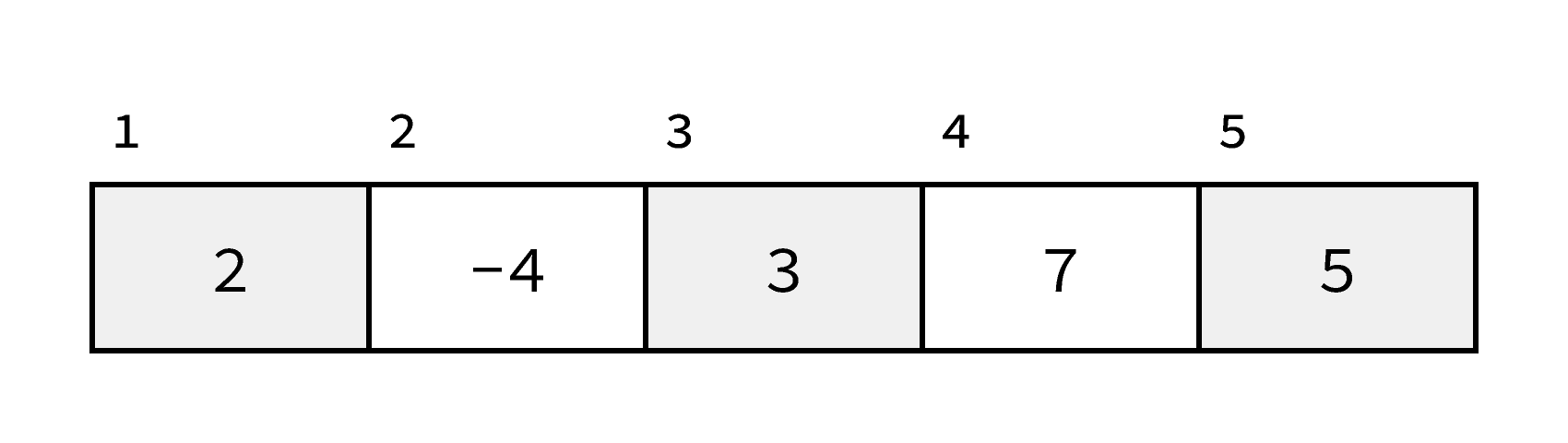On the array above, the algorithm should run and leave total set to 13.

# Arrays

Array algorithms frequently have multiple inputs. Construct an algorithm below so that, when the algorithm finishes, the assignable variable count will contain the number of values in the array A that are at least as big as x.

# Arrays

In this chapter, you’ve learned the basic tools that computer scientists use to talk about algorithms. In the next chapter, you’ll use these tools to explore basic algorithms that search and manipulate arrays.

The basics of pseudocode are worth learning, not just because algorithms are interesting, but because these basics are the foundation of almost every programming language! Commands, conditionals, while and for loops, assignable variables, and arrays are fundamental parts of almost every programming language.

# Arrays

×